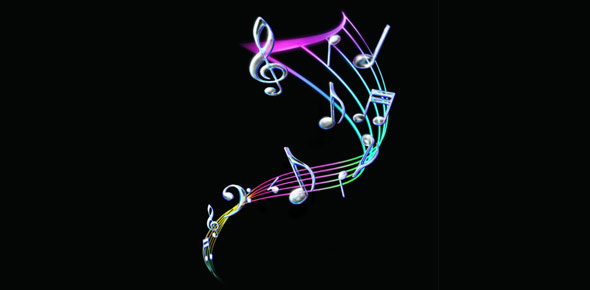# Key Signatures For Music Theory

12 Questions | Total Attempts: 239SettingsMajor and Minor key signatures

• 1.
What's the key signature of G major?
• A.

2 sharps

• B.

1 sharp

• C.

3 flats

• D.

3 sharps

• 2.
What's the key signature of B major?
• A.

3 flats

• B.

3 sharps

• C.

4 flats

• D.

2 flats

• 3.
What's the key signature of D major?
• A.

2 sharps

• B.

5 sharps

• C.

1 flat

• D.

5 flats

• 4.
What's the key signature of Db major?
• A.

2 flats

• B.

2 sharps

• C.

5 flats

• D.

4 flats

• 5.
What's the key signature of C# Major?
• A.

No sharps or flats

• B.

3 sharps

• C.

6 flats

• D.

7 sharps

• 6.
What's the key signature of Cb major?
• A.

6 flats

• B.

7 flats

• C.

6 sharps

• D.

7 sharps

• 7.
What's the key signature of Ab major?
• A.

4 flats

• B.

1 sharp

• C.

5 sharps

• D.

3 flats

• 8.
What's the key signature of Gb major?
• A.

7 sharps

• B.

6 flats

• C.

2 flats

• D.

5 sharps

• 9.
What's the key signature of A major?
• A.

2 sharps

• B.

4 sharps

• C.

5 sharps

• D.

3 sharps

• 10.
What's the key signature of F# major?
• A.

4 flat

• B.

3 sharps

• C.

6 sharps

• D.

2 flats

• 11.
What's the key signature of F major?
• A.

1 flat

• B.

3 sharps

• C.

2 flats

• D.

6 flats

• 12.
What's the key signature of E major?
• A.

4 sharps

• B.

2 flats

• C.

5 flats

• D.

4 flats

Related Topics